Beranda CIVIL ENGINEERING PROGRAM ROBOT STRUCTURAL Response Spectrum Analysis and Robot Structural

# Response Spectrum Analysis and Robot Structural

771
0
Details
 Language English Duration 11 Mins Format .MP4 Size 117 Mb
In this is video, Pat Tessaro walks you through the process of running a response spectrum analysis (often used to simulate earthquakes), explaining items such as mass participation while performing the analysis.

Theoretical basis for response spectrum calculations in spectral analysis
Understand that the method of determining response spectrum Sa=Sa(T) for a given diagram of accelerations F(t), where T refers to period, Sa refers to acceleration spectrum, t refers to time, while F(t) is a given time analysis function.

This is useful if in comparing the seismic responses obtained with the use of both spectral analysis and time analysis. The curve of response spectrum Sa=Sa(T), that overlaps exactly with the given function F(t) of time analysis (saving the acceleration diagram) should be ascribed.

You can analyze the dynamic structure response using the method of response spectrum (spectral analysis) for the current acceleration diagram. The pseudo-spectral acceleration is the maximum acceleration of a single degree of freedom due to the given diagram of acceleration.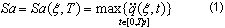where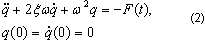Tp – recorded duration of an earthquake, ω = 2π/T – pulsation for Tmin ≤ T ≤ Tmax, ξ- damping parameter (expressed as a percentage of the critical damping), F(t) – given acceleration diagram.

In Figure 1, it is assumed, that the time analysis function F(t) is given. The sequence of Cauchy problems (2) for T = T1 < T2 < … < Tnpoints will be integrated numerically, where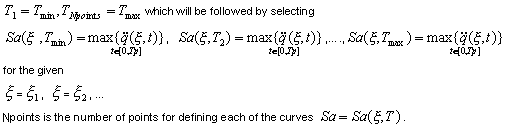The response spectrum is presented in Figure 2.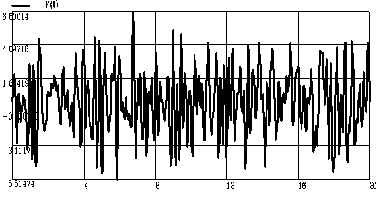Figure 1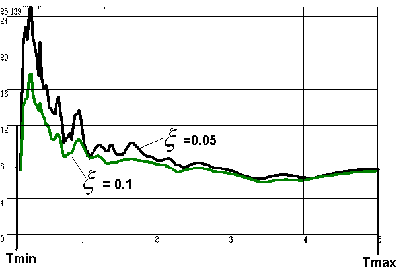Figure 2